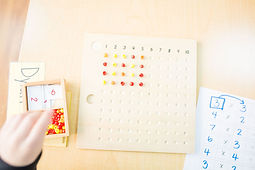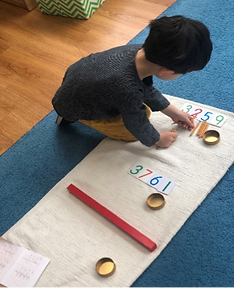top of page

math program

The HMS Montessori classroom and our math program is designed for the child's natural love for numbers and to facilitate a strong understanding of numerical concepts. The materials are presented sequentially, when an individual child is ready to understand the concepts, and  move through the curriculum at his or her own pace. Much of the Montessori curriculum is based on giving children exposure to concrete materials first, then giving them incremental opportunities to work to more abstract concepts.  This is no different when it comes to math.

Every material has its place. Each work on the shelves is presented to the child in ordered steps, so that they can do it accurately. The materials are arranged on the shelves from easiest to most difficult. This precision and order signature to the Montessori environment puts your child at ease to succeed. Gradually, as learning progresses and concepts are grasped your child will move from hands-on materials to performing mathematical functions abstractly.# "Children display a universal love of mathematics, which is par excellence the science of precision, order, and intelligence"Dr Maria Montessori

toddler years (ages 18 mo - 3 yrs)

Montessori math materials are beautifully designed for the child to “see” and “feel” concepts such as numeration, quantity, place value, fractions, and operations. This strong conceptual understanding serves as a foundation for later problem-solving and abstraction. Children will develop an awareness of numbers and basic math operations. The toddler uses hands-on materials for learning concrete math concepts. The toddler begins to understand number concepts, both quantity and symbol and the meaning of zero. The child also begins to understand the language used in performing simple single-digit addition.

primary years (ages 3-6 yrs)

Mathematical studies at the preschool level include numeration, decimal systems, computation, abstraction and memory work, fractions, money, time, measurement, geometry, and mathematical concepts.  Even before a child is able to count, they experience the skill of using materials like Sandpaper numbers, which teach children how to correctly form each number to develop readiness for writing them on paper.  When a child is ready to learn about basic operations, there are plenty of materials to support them.

kindergarten (ages 5-6 yrs)

The Kindergarten year is the one in which the concrete math instruction of the previous years comes to fruition. The children are now incorporating all of the concepts, from learning names for quantities one through ten to exchanging numbers. While teaching the decimal system, formation of numbers, and operations, concrete materials are consistently utilized, enabling the child to visualize and handle the materials, thus noting the differences in numerical value. As the hand is the child’s best teacher, he teaches himself the concepts needed to progress through math while using the Montessori math materials. After a multitude of exercises in the practice of math, the abstract begins to be introduced, in the form of recording answers. This is an important step in the gradual process of handling materials. The child now has the tools needed to progress to higher math of an abstract nature, with the understanding of basic mathematical concepts internalized.

math progression demonstrated#### sandpaper numerals

When a child works with the sandpaper numerals the introductory lesson is much the same as the sandpaper letters. The 3 period lesson is also used here; this is 7, show me number 7 and what number is this? This child is first introduced to a number, associates the symbol name and finally recalls it from memory.#### teens board

The teens board is the next stop when working off the math shelf. The child is introduced to place value. We always start with the tens place and introduce a teen number must start with ten, then the child will add a unit card to construct a tee number. The child then begins to understand that the tens and units become one number.#### loose & fixed quantity

After working with the sandpaper numerals we move on to teaching the child the symbol represents both a fixed and loose quantity. Here is where the child begins counting aloud and matching the colored beads to its appropriate symbol. Next, we move on to counting loose counters and once again matching to its correct number.#### solving equations

Once the child has a sound understanding of numerals and their quantities they will begin walking along their mathematical path towards problem solving. Here is where the child starts addition using manipulatives, from simple to complex. We use the term together with, rather than addition, to make this abstract concept more concrete.## child up for a lifetime of learning.